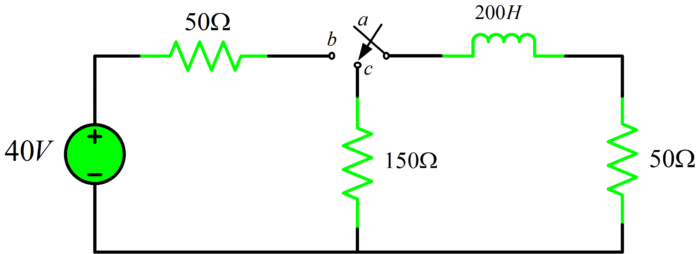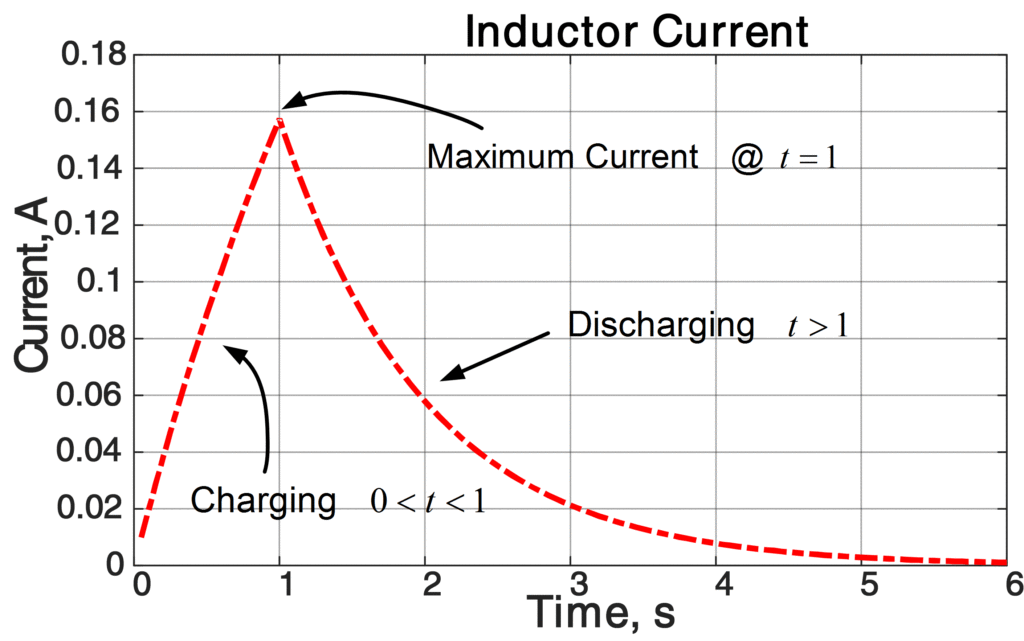# RL Circuit Charging Discharging | Matlab

Want create site? Find Free WordPress Themes and plugins.

In this tutorial, we will see an inductor current behavior in an RL Circuit using Matlab.

For the simplified RL circuit demonstrated below, an electric current flowing through an inductor is zero initially. At t = 0, the switch actuated from location a to b, where it stayed for 1 s. After 1s, the switch prompted from location b to location c, where it rested indefinitely. Draw the inductor current against time.Simple RL Circuit

In order to calculate charging current, will use the following relation:

Inductor Charging Current Equation$i(t)=\frac{{{V}_{s}}}{R}\left( 1-{{e}^{-\left( \frac{R}{L}t \right)}} \right)$

For 0<t<1, we will use above mentioned equation to find the current through an inductor

$i(t)=0.4\left( 1-{{e}^{-\left( \frac{t}{{{\tau }_{1}}} \right)}} \right)$

Where

${{\tau }_{1}}=\frac{L}{R}=\frac{200}{100}=2s$

Now, we will find the maximum current at t=1 using the same formula:

$i(t)=0.4\left( 1-{{e}^{-0.5}} \right)={{I}_{\max }}$

We will use the following formula to calculate the discharging current.

Inductor Discharging Current Equation$i(t)={{I}_{\max }}{{e}^{-\left( \frac{t}{\tau } \right)}}$

Now, for t>1, we will use above mentioned equation to find the discharging current

$i(t)={{I}_{\max }}{{e}^{-\left( \frac{t-0.5}{{{\tau }_{2}}} \right)}}$

Where

${{\tau }_{2}}=\frac{L}{R}=\frac{200}{200}=1s$

Now, let’s plot i(t) using Matlab and see inductor current behavior under charging and discharging.

## Inductor Charging and Discharging analysis with Matlab


clear all;close all;clc
% Inductor Current Calculation in an RL Circuit
Tau_1 = 200/100; % Charging Time Constant
for k=1:20
t(k) = k/20; % Loop for time (0&lt;t&lt;=1)
i(k) = 0.4*(1-exp(-t(k)/Tau_1)); % Charging Current Calculation using Formula mentioned in text for 0&lt;t&lt;1
end
i_max = i(20); %Maximum Current @ t=1s
Tau_2 = 200/200; % Discharging Time Constant
for k = 21:120
t(k) = k/20; % Loop for time (t&gt;1)
i(k) = i_max*exp(-t(k-20)/Tau_2) % Discharging Current Calculation using Formula mentioned in text for t&gt;1
end
%% Plotting the Current
plot(t,i,'r')
axis([0 6 0 0.18]) %Manual Adjustment to Limit the axis
title('Inductor Current')
xlabel('Time, s')
ylabel('Current, A')



ResultsDid you find apk for android? You can find new Free Android Games and apps.